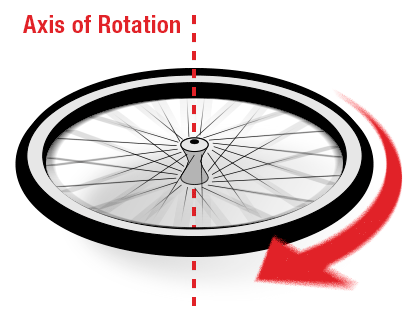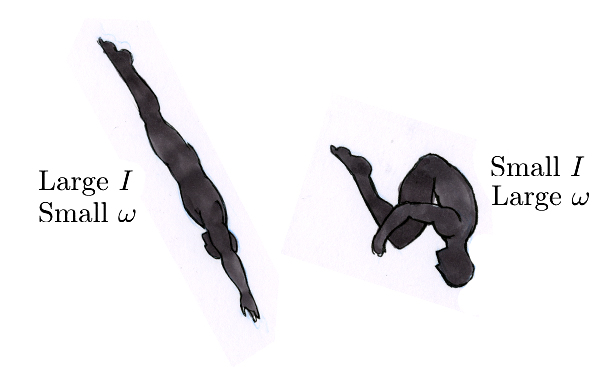# Rotation Study Guide

Studying rotation in objects requires you to understand a number of concepts such as angular velocity, angular momentum, and moment of inertia.

Table of content

### INTRODUCTION

Your friend is having a pool party, and everyone is challenging you to a diving contest! You’re great at performing fancy diving tricks for a long time and have the know-how to perform multiple flips in one dive successfully. You may not know the science, but you are aware that you will have to tuck your arms and knees as close to the chest as possible to spin faster.

What is the principle of physics that makes this work? This chapter in the rotation could just be what you need to study to further impress your friends at the next pool party! 😀Source

### WHAT IS ROTATION?

When objects rotate or are said to have a rotational motion, all the particles in the object move in a circular path around an axis of rotation. For example, think of a bicycle wheel that rotates about the axle as the axis of rotation. This is rotational motion in its simplest form. Other examples include rotation of the earth about its axis, rotation of ceiling fans, and merry-go-rounds!Source

Like linear motion, rotational motion has physical attributes such as angular velocity, moment of inertia, angular momentum, and torque.

### 1. ANGULAR VELOCITY

Angular velocity (ω) is defined as the angular displacement of an object with respect to time. If θ is the angular displacement in radians and t is the change in time, then the mathematical expression of angular displacement is given by:

``````                   Angular Velocity = ω = Δθ/Δt
``````

It is measured in the units radians/second

### 2. MOMENT OF INERTIA

The moment of inertia (I) measures the resistance offered by a body to rotation. The mathematical expression for moment of inertia is:

``````                           I= Mr²
``````

M is the mass of the rotating object r is the distance to the axis of rotation Unit is kg m2 If you observe the mathematical relation, you’ll understand that the moment of inertia is greater when the mass is distributed far from the center of rotation.

### 3. ANGULAR MOMENTUM

Like linear momentum, angular momentum measures how much motion is contained in the rotating body. Mathematically it is expressed as:

``````                          L = I ω
``````

L is the angular momentum I is the moment of inertia ω is the angular velocity. Unit is Kg.m2.s-1

### CONSERVATION OF ANGULAR MOMENTUMSource

Now for the fun pool part of rotational physics! According to the law of conservation of angular momentum, the total momentum of a rotating system never changes. When your arms and legs are stretched out during a dive, your moment of inertia is high, and angular velocity (front flip rotation) is low.

When you bring your arms and legs close to your chest, the moment of inertia decreases, and the angular velocity increases to maintain momentum, making you spin like a champ!

## CONCLUSION:

• An object is in rotational motion; all the particles move in a circular path around an axis of rotation.
• Angular velocity (ω) is defined as the angular displacement of an object with respect to time.
• The moment of inertia (I) measures the resistance offered by a body to rotation.
• Angular momentum gives a measure of how much motion is contained in the rotating body.

### FAQs:

1. What is rotation?

To explain rotation in a sentence, it is the movement of an object in a circular path about an axis of rotation.

2. What is a rotation example?

Rotation of bicycle wheel and rotation of the earth about its axis.

We hope you enjoyed studying this lesson and learned something cool about Rotation! Join our Discord community to get any questions you may have answered and to engage with other students just like you! Don't forget to download our App to experience our fun, VR classrooms - we promise, it makes studying much more fun! 😎

## SOURCES:

1. 8.1 Rotational Motion. https://flexbooks.ck12.org/cbook/ck-12-physics-flexbook-2.0/section/8.1/related/lecture/rotational-motion/. Accessed 27 Jan 2022.
2. 8.2 Angular Momentum. https://flexbooks.ck12.org/cbook/ck-12-physics-flexbook-2.0/section/8.2/primary/lesson/angular-momentum-physics-intermediate/. Accessed 27 Jan 2022.
3. Rotational Motion (Physics): What is it & Why it Matters. https://sciencing.com/rotational-motion-physics-what-is-it-why-it-matters-13721033.html. Accessed 27 Jan 2022.
4. Angular Velocity. https://byjus.com/physics/angular-velocity/. Accessed 27 Jan 2022.